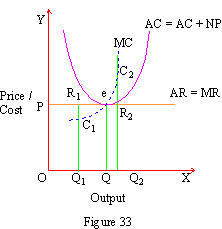Home MonkeyNotes Printable Notes Digital Library Study Guides Study Smart Parents Tips College Planning Test Prep Fun Zone Help / FAQ How to Cite New Title Request

 10.2 Competitive Equilibrium (A) MR = MC rule: Equilibrium of a firm is a condition where profits are maximized. The analytical condition of equilibrium is stated as a point of equality between Marginal Revenue and Marginal Cost. It ensures the profit as Profitmax = TR - TCmax where MR = MC i.e. the difference between Total Revenue and Total Cost. (TR - TC) is maximized automatically when this condition is satisfied. This MR = MC rule is equally applicable to a competitive or monopolistic or oligopolistic or any other form of the market. This can be explained with the help of a figure.In Figure 33, P - AR = MR is the demand or Average and Marginal Revenue curve. AC is the Average Cost curve and MC the Marginal Cost curve. Point e is the point of intersection of AC and MC; hence at the minimum point on AC there is equality between MR and MC. Therefore e is the point of equilibrium where profits are maximized. At the equilibrium point market price is P and output produced is Q. For any other output level, profits cannot be further increased. For a smaller output level Q1 the point on the Marginal Revenue curve R1 is above the point e1 on the Marginal Cost curve. Hence to the left of point e so long as MR > MC a firm can gain more profits by increasing output and by moving in the direction of point e. On the other hand, if a firm tries to produce a larger output Q2 then Marginal Cost C2 exceeds Marginal Revenue R2 (MC > MR) and the firm makes some losses. These losses can be avoided only by restricting output and by moving in the direction of point e. Hence we conclude that e is the point of equilibrium which alone can help to maximize the profits of a firm. Note that the firm is operating under competitive market conditions. It earns only normal profits which are included in the average cost of the production curve AC. We have therefore marked AC as AC + NP curve. In a competitive market, a firm will be in equilibrium at a point e where all the four variables are equal. MR = MC = AR = AC. A firm in such equilibrium earns only normal profit. AC = AC + NP Index 10.1 - Features of Competition10.2 - Competitive Equilibrium10.3 - Short Run Equilibrium10.4 - Long Run Equilibrium10.5 - Exit or Shutdown Point Chapter 11
 Search: All Products Books Popular Music Classical Music Video DVD Toys & Games Electronics Software Tools & Hardware Outdoor Living Kitchen & Housewares Camera & Photo Cell Phones Keywords: